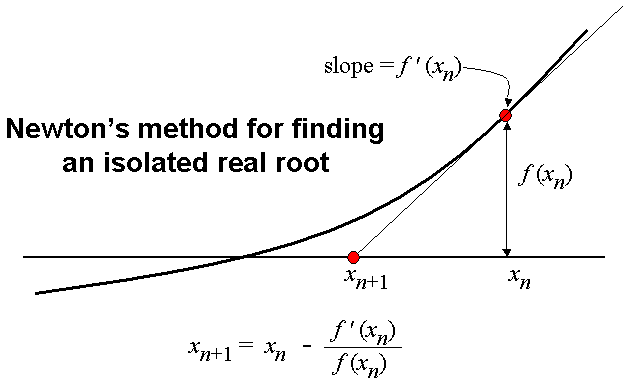# C Program for Newton-Raphson Method

Newton-Raphson Method, is a Numerical Method, used for finding a root of an equation.
The method requires the knowledge of the derivative of the equation whose root is to be determined. So we would have to enter that manually in our code.Newton-Raphson Method may not always converge, so it is advisable to ask the user to enter the maximum number of iteration to be performed in case the algorithm doesn’t converge to a root. Moreover, since the method requires division by the derivative of the function, one should add a condition that prevents division by zero.

The following is a simple version of the program that finds the root, and tabulates the different values at each iteration. Just like any other numerical method bisection method is also an iterative method, so it is advised to tabulate values at each iteration.

### PROGRAM(Simple Version):

```/******************************
****NEWTON RAPHSON METHOD*****
2017 (c) Manas Sharma - https://bragitoff.com
******************************/
#include<stdio.h>
#include<math.h>

/*Function whose root is to be determined*/
double f(double x){
return 3*x+sin(x)-exp(x);
}

/*Derivative of the function whose root is to be determined*/
double df(double x){
return 3-cos(x)-exp(x);
}

int main(){
double x,eps,x1;
int maxSteps;
printf("Enter the initial guess:\n");
scanf("%lf",&x1);
printf("Enter the desired accuracy:\n");
scanf("%lf",&eps);
printf("Enter the max. number of steps:\n");
scanf("%d",&maxSteps);
int iter=1;
/*Newton-Raphson Method begins that tabulates the various values at each iteration*/
printf("____________________________________________________________________________________\n");
printf("x\tf(x)\t\tf'(x)\t\tx1\t\t|x-x1|\t\tf(x1)\n");
printf("____________________________________________________________________________________\n");
do{
x=x1;
/* IF-Condition to prevent division by zero[To be done: Check for infinite values too]*/
if(fabs(df(x))>=0.000000001&&df(x)!=NAN){
/*New value of x using the NR Expression */
x1=x-f(x)/df(x);
printf("%d.\t%lf\t%lf\t%lf\t%lf\t%lf\n",iter,f(x),df(x),x1,fabs(x-x1),f(x1));
iter++;
}
else{
printf("Sorry! The slope is 0 for one of the iterations.\n NR Method failed.\n");
return 0;
}

}while(fabs(x-x1)>=eps&&iter<=maxSteps);
printf("_______________________________________________________________________________________\n\nOne of the roots of the given equation is:\n\n%lf\n\n\n",x1);

}
```

The better version of the above code uses a function called ‘rootNR’ to perform the NR task and return the root.
However, this function won’t tabulate the values at each iteration.
So in the following program I have also provided another function called ‘printNR’ that would return the root as well as print the various values at each iteration.

### PROGRAM(Better Version):

```/******************************
****NEWTON RAPHSON METHOD*****
2017 (c) Manas Sharma - https://bragitoff.com
******************************/
#include<stdio.h>
#include<math.h>

/*Function whose root is to be determined*/
double f(double x){
return x*x*x-27;
}

/*Derivative of the function whose root is to be determined*/
double df(double x){
return 3*x*x;
}

/*Function that returns the root from Newton-Raphson Method*/
double rootNR(double f(double x),double df(double x),double x1,double eps,double maxSteps){
double x;
int i=1;
do{
x=x1;
if(fabs(df(x))>=0.000000001){
x1=x-f(x)/df(x);
i++;
}
}while(fabs(x-x1)>=eps&&i<=maxSteps);
return x1;
}

/*Newton-Raphson Method Function that tabulates the values at each iteration*/
double printNR(double f(double x),double df(double x),double x1,double eps,double maxSteps){
double x;
int iter=1;
printf("___________________________________________________________________________________________________\n");
printf("iter\tx\t\tf(x)\t\tf'(x)\t\tx1\t\t|x-x1|\t\tf(x1)\n");
printf("___________________________________________________________________________________________________\n");
do{
x=x1;
if(fabs(df(x))>=0.000000001){
x1=x-f(x)/df(x);
printf("%d.\t%lf\t%lf\t%lf\t%lf\t%lf\t%lf\n",iter,x,f(x),df(x),x1,fabs(x-x1),f(x1));
iter++;
}
}while(fabs(x-x1)>=eps&&iter<=maxSteps);
return x1;
}
main(){
double x,eps,x1;
int maxSteps;
printf("Enter the initial guess:\n");
scanf("%lf",&x);
printf("Enter the desired accuracy:\n");
scanf("%lf",&eps);
printf("Enter the max. number of steps:\n");
scanf("%d",&maxSteps);
printf("__________________________________________________________________________________________________\n\nOne of the roots of the given equation is:\n\n%lf\n\n\n",printNR(f,df,x,eps,maxSteps));

}

```

### Related Posts:## 2 thoughts on “C Program for Newton-Raphson Method”

1. Thanks, you help me in my college jobs.

2. thanks, i need this program